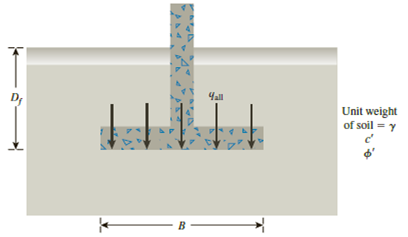# Redo Problem 16.4 using the modified general ultimate bearing capacity Eq. (16.31). 16.1 A continuous footing is shown in Figure 16.17. Using Terzaghi’s bearing capacity factors, determine the gross allowable load per unit area ( q all ) that the footing can carry. Assume general shear failure. Given: γ = 19 kN/m 3 , c ′ = 31kN/m 2 , ϕ ′ = 28 ° , D f = 1.5 m, B = 2 m, and factor of safety = 3.5. Figure 16.17 16.4 Redo Problem 16.1 with the following: γ = 16.5 kN/m 3 , c u = 41 kN/m 3 , ϕ ′ = 0 , D f = 1.5 m, and factor of safety = 5.### Principles of Geotechnical Enginee...

9th Edition
Braja M. Das + 1 other
Publisher: Cengage Learning
ISBN: 9781305970939### Principles of Geotechnical Enginee...

9th Edition
Braja M. Das + 1 other
Publisher: Cengage Learning
ISBN: 9781305970939

#### Solutions

Chapter
Section
Chapter 16, Problem 16.8P
Textbook Problem

## Redo Problem 16.4 using the modified general ultimate bearing capacity Eq. (16.31).16.1 A continuous footing is shown in Figure 16.17. Using Terzaghi’s bearing capacity factors, determine the gross allowable load per unit area (qall) that the footing can carry. Assume general shear failure. Given: γ = 19 kN/m3, c′ = 31kN/m2, ϕ ′ = 28 ° , Df = 1.5 m, B = 2 m, and factor of safety = 3.5.Figure 16.1716.4 Redo Problem 16.1 with the following: γ = 16.5 kN/m3, cu = 41 kN/m3, ϕ ′ = 0 , Df = 1.5 m, and factor of safety = 5.

Expert Solution
To determine

Find the gross allowable load per unit area (qall) that the footing can carry using modified general ultimate bearing capacity.

### Explanation of Solution

Given information:

The unit weight of the soil γ is 16.5kN/m3.

The value of uniformity coefficient cu is 41kN/m3.

The soil friction angle ϕ is 0°.

The depth of foundation Df is 1.5 m.

The width of footing B is 2 m.

The factor of safety Fs is 5.

Calculation:

Determine the depth factor λcd using the relation.

λcd=1+0.4(DfB)

Substitute 1.5 m for Df and 2 m for B.

λcd=1+0.4(1.52)=1.3

Determine the depth factor λqd using the relation.

λqd=1+2tanϕ(1sinϕ)2(DfB)

Substitute 0° for ϕ, 1.5 m for Df, and 2 m for B.

λqd=1+2tan0°(1sin0°)2(1.52)=1.0

Determine the ultimate bearing capacity of the soil (qu) using the relation.

qu=cuNcλcsλcdλci+qNqλqsλqdλqi+12γBNγλγsλγdλγi=cuNcλcsλcdλci+γDfNqλqsλqdλqi+12γBNγλγsλγdλγi (1)

Here, λcs,λqs,andλγs are the shape factors, Nc is the contribution of cohesion, λci,λqi,andλγi are the inclination factors, Nq is the contribution of surcharge, and Nγ is the contribution of unit weight of soil

### Want to see the full answer?

Check out a sample textbook solution.See solution

### Want to see this answer and more?

Bartleby provides explanations to thousands of textbook problems written by our experts, many with advanced degrees!

See solution

Find more solutions based on key concepts
Show solutions
Describe the basic ideas of probability.

Engineering Fundamentals: An Introduction to Engineering (MindTap Course List)

What is CAM software?

Precision Machining Technology (MindTap Course List)

Define the purpose of the final drive gears.

Automotive Technology: A Systems Approach (MindTap Course List)

Discuss the importance of data models.

Database Systems: Design, Implementation, & Management

Same as Problem 9.8-3, but use ASD.

Steel Design (Activate Learning with these NEW titles from Engineering!)

Why might organizations be willing to take on the risk of BYOD?

Network+ Guide to Networks (MindTap Course List)

Dead Battery While traveling for business, you realize that you forgot to bring the battery charger for your la...

Enhanced Discovering Computers 2017 (Shelly Cashman Series) (MindTap Course List)

What can happen if too much power is being carried by a cable?

Welding: Principles and Applications (MindTap Course List)

If your motherboard supports ECC DDR3 memory, can you substitute non-ECC DDR3 memory?

A+ Guide to Hardware (Standalone Book) (MindTap Course List)